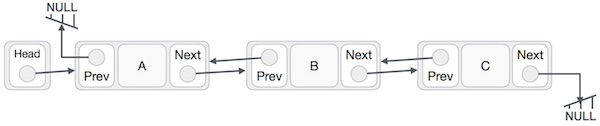# Data Structure - Doubly Linked List

Doubly Linked List is a variation of Linked list in which navigation is possible in both ways, either forward and backward easily as compared to Single Linked List. Following are the important terms to understand the concept of doubly linked list.As per the above illustration, following are the important points to be considered.

• Doubly Linked List contains a link element called first and last.

• Each link carries a data field(s) and two link fields called next and prev.

• The last link carries a link as null to mark the end of the list.

## Basic Operations

Following are the basic operations supported by a list.

• Insertion − Adds an element at the beginning of the list.

• Deletion − Deletes an element at the beginning of the list.

• Insert Last − Adds an element at the end of the list.

• Delete Last − Deletes an element from the end of the list.

• Insert After − Adds an element after an item of the list.

• Delete − Deletes an element from the list using the key.

• Display forward − Displays the complete list in a forward manner.

• Display backward − Displays the complete list in a backward manner.

## Insertion Operation

Following code demonstrates the insertion operation at the beginning of a doubly linked list.

### Example

```//insert link at the first location
void insertFirst(int key, int data) {

struct node *link = (struct node*) malloc(sizeof(struct node));

if(isEmpty()) {
} else {
}

//point it to old first link

//point first to new first link
}
```

## Deletion Operation

Following code demonstrates the deletion operation at the beginning of a doubly linked list.

### Example

```//delete first item
struct node* deleteFirst() {

last = NULL;
} else {
}

}
```

## Insertion at the End of an Operation

Following code demonstrates the insertion operation at the last position of a doubly linked list.

### Example

```//insert link at the last location
void insertLast(int key, int data) {

struct node *link = (struct node*) malloc(sizeof(struct node));

if(isEmpty()) {
} else {